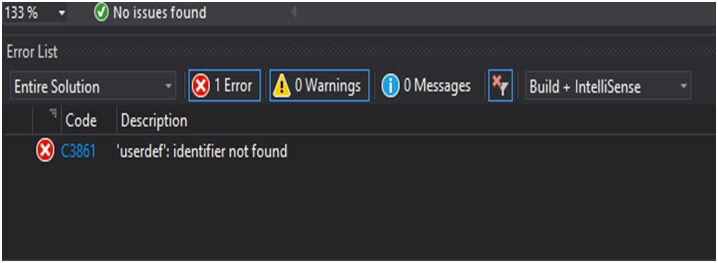# Function protocol and function definition in C++ programming

In this tutorial, we are going to learn about the function protocol and function definition in C++ programming language.
Submitted by Aditi S., on June 02, 2019

Function prototype and function definition in C++ programming come into play while working with user-defined functions. User-defined functions are functions defined by the user as per the requirement of the program or to solve a given set of queries.

There are two major parts of a user-defined function: 1) the function header which contains the Return type, Name and Parameter list of a function and 2) the function body that contains the set of statements or operations required needed to be carried out during the call of the function. The function header and the body of the function together are called as the function definition.

Example of function definition:

```void userdef()
{
cout <<"User defined function \n";
}
```

When a function is defined after the main function the compiler fails to recognize the function during its call when the program is being compiled, an error is displayed.To prevent this a function prototype is defined before the main function. A function prototype contains only the function header.

```void userdef();
//or
void userdef(void);
```

### C++ program to show function definition and prototype

```//C++ program to demonstrate example of
//function prototype and function definition
//(adding two numbers using user define function)

#include <iostream>
using namespace std;

//Function Prototype
int sum(int a, int b);

//main function
int main()
{
int x, y;
int result;
cout << "Enter two numbers: ";
cin >> x >> y;
result = sum(x, y);
cout << "Sum of numbers is: " << result << "\n";
return 0;
}

//Function Definition
int sum(int a, int b)
{
int z;
z = a + b;
return z;
}
```

Output

```Enter two numbers: 36 24
Sum of numbers is: 60
```

Top MCQs

Languages: » C » C++ » C++ STL » Java » Data Structure » C#.Net » Android » Kotlin » SQL
Web Technologies: » PHP » Python » JavaScript » CSS » Ajax » Node.js » Web programming/HTML
Solved programs: » C » C++ » DS » Java » C#
Aptitude que. & ans.: » C » C++ » Java » DBMS
Interview que. & ans.: » C » Embedded C » Java » SEO » HR
CS Subjects: » CS Basics » O.S. » Networks » DBMS » Embedded Systems » Cloud Computing
» Machine learning » CS Organizations » Linux » DOS
More: » Articles » Puzzles » News/Updates